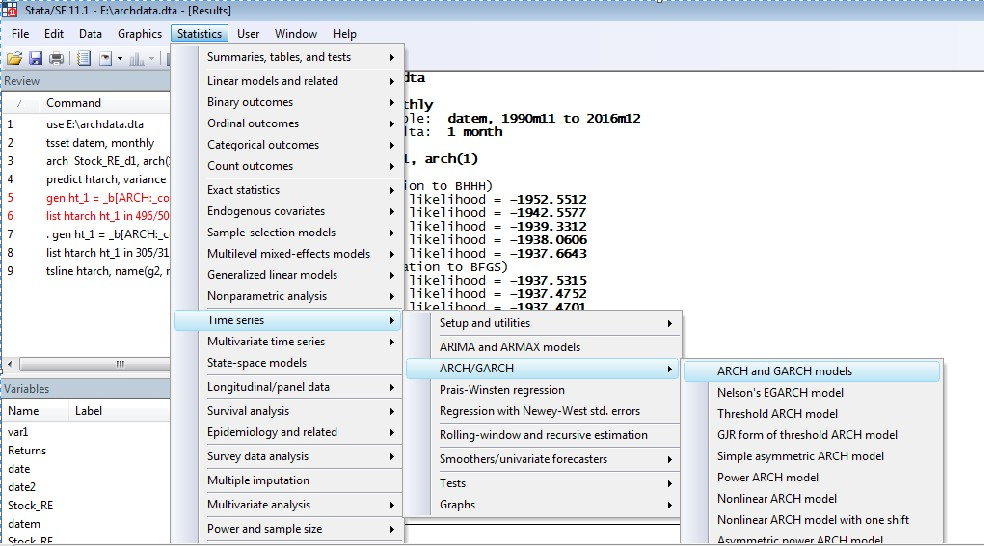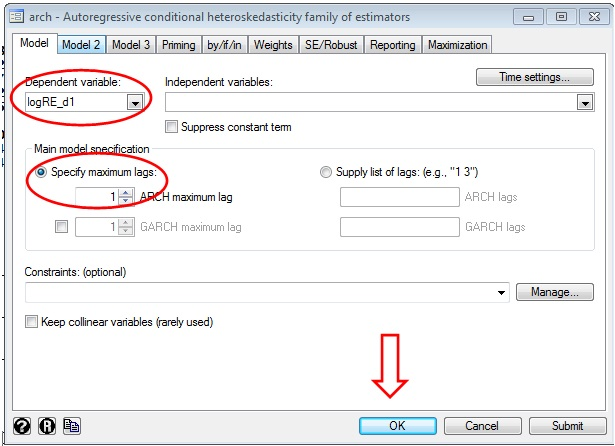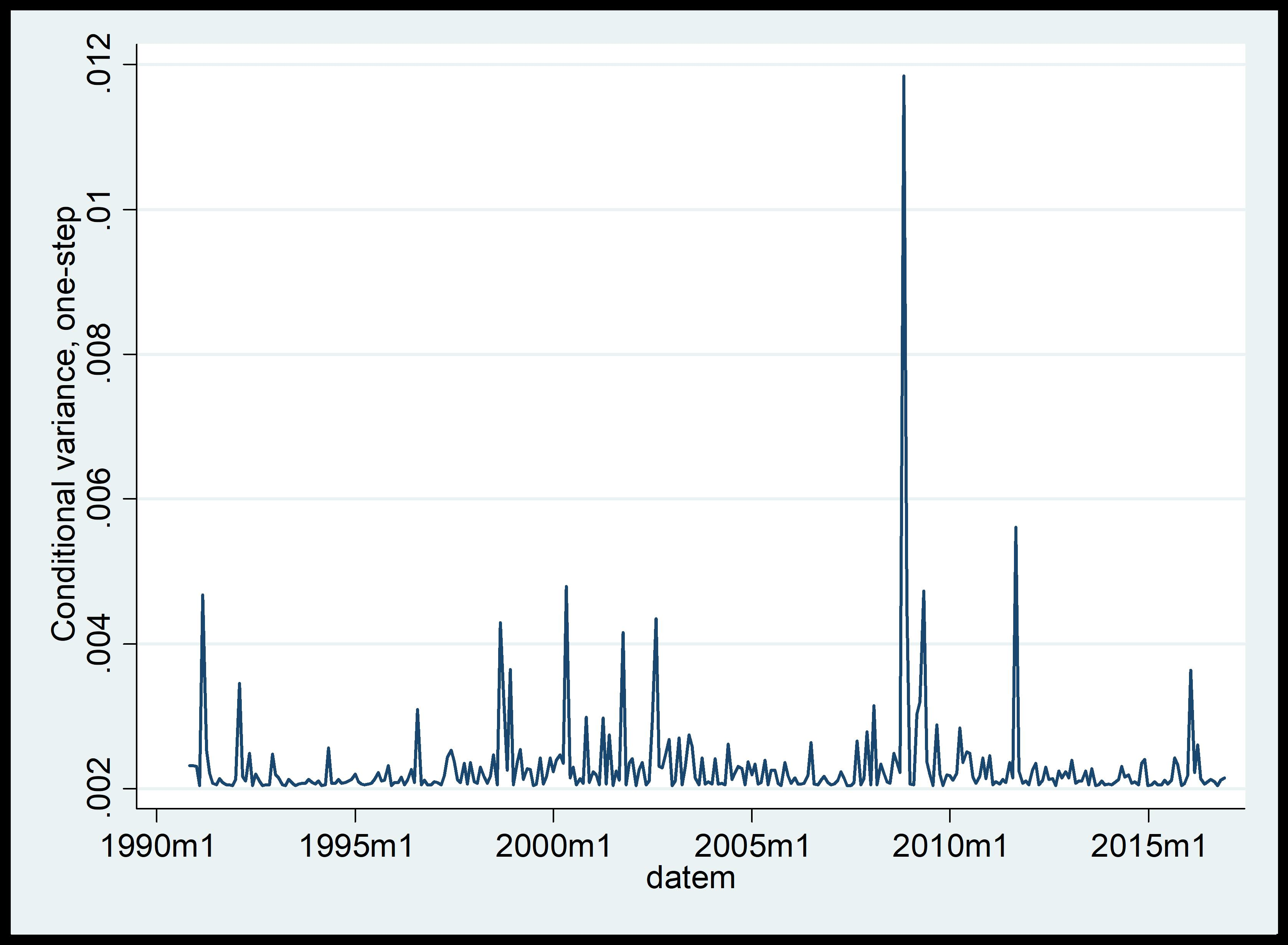# ARCH model for time series analysis in STATA

The previous article showed how to initiate the AutoRegressive Conditional Heteroskedasticity (ARCH) model on a financial stock return time series for period 1990 to 2016. It showed results for stationarity, volatility, normality and autocorrelation on a differenced log of stock returns. The article concluded that the series has an ARCH effect. In continuation, this article presents the ARCH model of the same series.

## Applying the ARCH model for time series with lag 1

In order to apply the ARCH model for time series, follow these steps in STATA:

1. Click on ‘Statistics’ in the output window.
2. Select ‘time series’.
3. Select ‘ARCH/GARCH’.
4. Click on ‘ARCH and GARCH models’.Figure 1: Pathway for ARCH model in STATA

A dialogue box named as ‘arch- Autoregressive conditional hetroskedasticity family of estimators’ will appear on STATA as shown in the figure below. Select two items; the concerned variable i.e. ‘logRE_d1’, and the number of lags, i.e. ‘1’.Figure 2: Dialogue box for ARCH model in STATA

Alternatively, use the below command for the ARCH model:

`arch  Stock_RE_d1, arch(1)`

## Results for lag 1

The results will appear. A list of iterations will appear showing nonlinear numerical optimization which was done behind the scenes. In this case, it helped maximize the likelihood function. The log-likelihood will increase as the iterations proceed. If the numerical optimization somehow fails, then an error message will appear just after the iterations. The parameter estimates follow the iteration summary.  Therefore, the average return is log 0.0103. The ARCH term’s t-ratio is statistically significant, however, the lag1 is insignificant.Figure 3: Results for ARCH model with lag 1 in STATA

## Applying ARCH for time series with lag 2

Since the result with 1 lag is insignificant, process the ARCH results once again with a greater number of lags, suppose 2.Figure 4: ARCH model with lag 2 in STATA

Alternatively, use the below command:

`arch  Stock_RE_d1, arch(2)`

## Results for lag 2

The results for the ARCH model with lag 2 will appear as shown in the figure below. Similar to the previous case, the log-likelihood will increase the iterations proceed. The parameter estimates to follow the iteration summary. Therefore, the average return is log 0.009. The ARCH term’s t-ratio is statistically significant and this time the lag2 is significant. Therefore, this ARCH model is best fitted.Figure 5: Results of ARCH model with lag 2 in STATA

Therefore, the ARCH model correctly examined the trend in the time series of stock returns.

## Predicting the variance of a series

It is also possible to predict the variance of this series to get a clarification about the swings invariance over the time. Use this command:

`predict Mtarch, variance`

‘Mtarch’ here is the name for variance of time series stock returns. The results for above command, however, will not appear in ‘Result’ window, but in ‘Data editor’ window of STATA. In order to examine the movement of a variance of Mtarch, generate time plot using this command:

`tsline Mtarch`

The result will appear as below.Figure 6: Time plots of predicted variances under ARCH model

Thus the above graph shows that there is a lot more volatility towards the year 2010.

This article demonstrated ARCH model. The next article reviews some extensions of the model such as Generalized Autoregressive Conditional Heteroskedasticity (GARCH) and Threshold- Generalized Autoregressive Conditional Heteroskedasticity (TGARCH).

### Saptarshi Basu Roy Choudhury

Senior Research Analyst at Project Guru
Saptarshi has done his M. Phil in International Trade and Development and Masters in Economics from Jawaharlal Nehru University, New Delhi. His academic interests include issues related to economics of climate change, regulation and contemporary trade theories. He has a keen interest in current affairs and likes to read and travel in his spare time.

#### Latest posts by Saptarshi Basu Roy Choudhury (see all)We are looking for candidates who have completed their master's degree or Ph.D. Click here to know more about our vacancies.